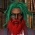Display replies flat, with oldest first Display replies flat, with newest first Display replies in threaded form Display replies in nested formKonstantin Pileckas (Vilnius University) “Solution of Leray's problem for the stationary Navier-Stokes equations in plane domains”by Egor Pifagorov - Sunday, 17 September 2017, 02:28 AM МИНИКУРС ЛАБОРАТОРИИ ИМ. ЧЕБЫШЕВА Konstantin Pileckas (Vilnius University) “Solution of Leray's problem for the stationary Navier-Stokesequations in plane domains” Лаборатория Чебышева, ауд. 413, 14-я линия В.О., 29. Расписание лекций:среда, 11 октября, 17:15;пятница, 13 октября, 17:15;суббота, 14 октября, 10:30 (2 лекции). In the series of lectures, we study the nonhomogeneous boundary value problem for the Navier-Stokes equations of the steady motion of a viscous incompressible fluid in arbitrary bounded multiply connected domain. We start from the classical results and then present in historical order more recent ones. Finally we prove the solvability of the nonhomogeneous boundary value problem for the Navier-Stokes equations in a bounded plane multiply connected domain under the sole necessary condition of zero total flux through the boundary. This result solves the problem formulated by Jean Leray more than 80 years ago (in 1933) and nowadays called Leray's problem. The essentially new ingredient in the proof is the Bernoulli Law for Sobelev space solutions of the Euler equations, which in turn is based on Morse-Sard theorem for Sobolev functions proved by J. Bourgain, M. Korobkov and J. Kristensen. We also present new results concerning Navier-Stokes equations in bounded axially symmetric three-dimensional domains as well as in unbounded domain.Приглашаются все желающие!